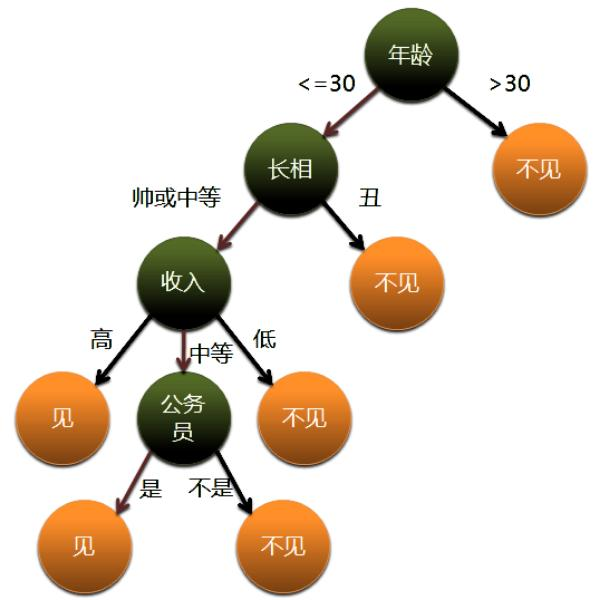# 比较排序的最优解log (N!)

= logN + log(N-1).....+log(1)

> = logN + log(N-1).....+log(N/2) >= (N/2)log(N/2)

= Ω(N log N)